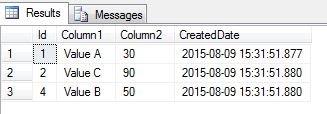# How to delete duplicate rows from a table in SQL Server

Here’s a question that probably most SQL Server DBAs or developers had to answer at some point in their career: how do you remove duplicate rows from a table? Maybe that duplicate data came in through an error in some ETL process or maybe it was an error in a front-end application that allowed end users to submit data multiple times. At any rate – the problem that caused duplicate data is now fixed and we have to cleanup the duplicates from our table. (This is also a perfect scenario / question for a SQL Server job interview because it will allow you to quickly tell how a candidate approaches SQL problems that are not exactly trivial – we’ll see why in a little bit.)

Let’s set up a table and insert some sample data into it:

```CREATE TABLE [dbo].[SampleData](
[Id] [int] IDENTITY(1,1) NOT NULL,
[Column1] [varchar](50) NOT NULL,
[Column2] [int] NOT NULL,
[CreatedDate] [datetime] NOT NULL,
CONSTRAINT [PK_SampleData] PRIMARY KEY CLUSTERED
(
[Id] ASC
)WITH (PAD_INDEX = OFF, STATISTICS_NORECOMPUTE = OFF, IGNORE_DUP_KEY = OFF, ALLOW_ROW_LOCKS = ON, ALLOW_PAGE_LOCKS = ON) ON [PRIMARY]
) ON [PRIMARY]

GO

ALTER TABLE [dbo].[SampleData] ADD  CONSTRAINT [DF_SampleData_CreatedDate]  DEFAULT (getdate()) FOR [CreatedDate]
GO

INSERT INTO SampleData (Column1, Column2) VALUES ('Value A', 30)
INSERT INTO SampleData (Column1, Column2) VALUES ('Value C', 90)
INSERT INTO SampleData (Column1, Column2) VALUES ('Value A', 30)
INSERT INTO SampleData (Column1, Column2) VALUES ('Value B', 50)
INSERT INTO SampleData (Column1, Column2) VALUES ('Value A', 30)
INSERT INTO SampleData (Column1, Column2) VALUES ('Value B', 50)
INSERT INTO SampleData (Column1, Column2) VALUES ('Value C', 90)
INSERT INTO SampleData (Column1, Column2) VALUES ('Value B', 50)
INSERT INTO SampleData (Column1, Column2) VALUES ('Value B', 50)

```

If we now look at data in the SampleData table this is what we’ll see:Values in the Id column are all different because that’s our identity column. Values in the CreatedDate column may or may not be different depending on how the INSERT statements were grouped together. As far as the other data columns it’s clear that we have a problem because we have some rows that are duplicates of others. What we want to do is to remove all duplicates for each combination of values and only keep the earliest one in each subset (we’ll define that as the one with the smallest Id value).

This is our problem. How do we go about removing those duplicate rows in the most efficient way as far as SQL Server is concerned?

Some SQL developers will look at this and their first instinct will be to come up with some sort of complex RBAR (Row By Agonizing Row) algorithm to deal with this … this will be especially true if these developers come from a non-SQL programming background. They’ll start running loops and cursors and their solution could get quite complex before you know it. That’s why I said this would be a good question to ask in a SQL Server job interview – if you see individual row processing as part of the solution you’ll probably want to think twice about that candidate.

What’s wrong with RBAR? To put it simply it goes against what SQL Server is best at. SQL Server will perform best and be most efficient when data can be processed as sets. Anytime we find ourselves having to process something row by row in Transact-SQL we’re most likely doing something wrong.

If we stop and think a little about the problem this is what we would like: we would like to be able to break up this data into subsets (one subset for each group of rows that share the same data values) and then for each such subset figure out the first row (the one we’d keep) and delete all the others.

The idea of working on subsets of data should immediately make us think of the various window functions in T-SQL … in particular ROW_NUMBER() which will be the one most useful to us in this situation. We’ll also make use of a CTE (common table expression) to make our code easier to follow.

Let’s create the following CTE that will divide our original data into subsets based on the combination of column values and label the rows for us so we can easily tell which one is the first one (the one we’d like to keep):

```WITH OrderedRows
AS
(
SELECT Id, Column1, Column2, CreatedDate,
ROW_NUMBER() OVER(PARTITION BY Column1, Column2 ORDER BY Id) AS RowOrder
FROM SampleData
)```

If we look at data returned by this CTE it will be clear what our next step should be:

`SELECT * FROM OrderedRows`How is data returned by the CTE different from our original view of the data? We see now that rows are grouped together based on values found in Column1 / Column2 and for each subset the new column RowOrder (obtained from the use of the ROW_NUMBER() function) will order the rows in that particular subset based on the value in the Id column (the row with the lowest Id value in each subset will always be given the value 1 by ROW_NUMBER).

Looking at this it’s clear now that in order to delete duplicates we just need to remove all rows from the CTE where the RowOrder value is greater than 1.

`DELETE FROM OrderedRows WHERE RowOrder > 1`

Let’s look at data in our SampleData table after the delete operation above was executed:All duplicate rows are now gone and we kept the earliest instance (the row with smallest Id) for each combination of Column1 / Column2. We did all this using native T-SQL functions that allowed us to work with data at the set level – no complex row by row processing.